### Static and flowing fluids

Static fluids

#### Problem:

What is the hydrostatic force on the back of Grand Coulee Dam if the water in the reservoir is 150m deep and the width of the dam is 1200m.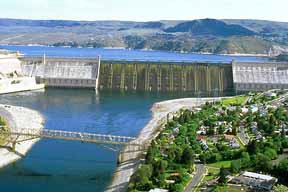Solution:

• Concepts:
The relationship between force and pressure
The pressure at a point below the surface of a liquid at rest in a constant gravitational field depends only on the depth d of that point and the pressure at the surface.
P = P0 + ρgd.
• Reasoning:
The water behind the dam at rest.
• Details of the calculation:
Let us denote the distance below the surface by y.  The pressure on the side of the dam facing the water at depth y is P0 + ρgy.  Here P0 is the atmospheric pressure (101 kPa) and ρ is the density of water.  The force on a surface element of width w and height dy at depth y is
dF = (P0 + ρgy)dA = (P0 + ρgy)wdy.
The total force on this side of the dam points outward and has magnitude
0150 dF = wP00150 dy + wρg∫0150 ydy = wP0150 + wρg1502/2.
(All quantities are measured in SI units.)
The total force on the side of the dam facing air is wP0150, inward.  The net force on the dam is F = wρg1502/2, outward.  With w = 1200m and ρ = 1000kg/m3, F = 1.32*1011N.

#### Problem:

A pipe of length 180 m, open on one end and closed on the other, lies at the bottom of a 200 m deep lake.  A light movable piston is placed inside the pipe.  The space between the closed end of the pipe and the piston is filled with air.  The piston is in equilibrium 20 m away from the closed end of the pipe.
The open end of the pipe is very slowly raised until the pipe is brought into the vertical position, its closed end resting at the bottom of the lake.
What is the height of the air column inside the vertical pipe?
Neglect the atmospheric pressure and assume the water temperature is the same throughout the lake.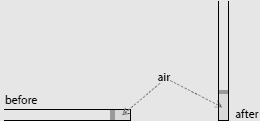Solution:

• Concepts:
The ideal gas law, pressure in a liquid as a function of depth
• Reasoning:
In equilibrium the air pressure in the pipe equals the water pressure, Pwater = ρwatergd, neglecting the atmospheric pressure.  Here d is the depth below the surface of the water.
• Details of the calculation:
Before: Pwater = ρwaterg*200 m = nkT/(A 20 m).
Here A is the cross-sectional area of the pipe.
nkT/A = ρwaterg*4000 m2 = constant.
After: ρwaterg*(200 m - h) = nkT/(A h).
nkT/A = ρwaterg*(200 m - h)h.
4000 m2 = (200 m - h)h.
h = (100 ± √6000) m.
h = (100 - 20*√15) m = 22.5 m.
Only the solution h = 100 - 20*√15 represents a stable equilibrium.
d(Pwater(h) - Pair(h))/dh must be positive for a stable equilibrium to exist.
d(Pwater - Pair)/dh = -ρwaterg + nkT/(Ah2) = ρwaterg*(4000 m/h2 - 1).
For h = (100 - 20*√15) m, d(Pwater - Pair)/dh is positive but for h = (100 + 20*√15) m it is negative.

Flowing fluids

#### Problem:

A cylindrical vessel of radius R = 0.1 m is filled with a liquid to a height of 0.5 m.  It has a capillary tube 0.15 m long and radius r = 0.0002 m fixed horizontally at its bottom.  Find the time in which the water level will fall to a height of 0.2 m.  Assume an ideal fluid in a frictionless environment.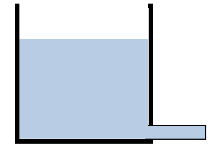Solution:

• Concepts:
Bernoulli's equation and the continuity equation
• Reasoning:
We have an ideal incompressible fluid
• Details of the calculation:
We introduce points B and C as shown in the figure below.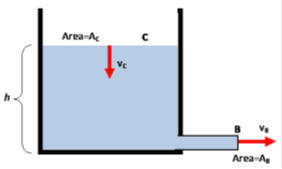The velocities at these two points are vC and vB.
The continuity equation yields vCAC = vBAB.
AC = πR2 = 0.0314 m2,  AB = πr2 = 1.3*10-7 m2.
vC = 4*10-6 vB.
The velocity of liquid at point C is much smaller than the velocity of the liquid at point B.
The pressure at points B and C is atmospheric pressure.  Bernoulli's equation therefore yields
ρgh + ρvC2/2 = ρvB2/2.
Since vC << vB then we have ρgh = ρvB2/2,  vB = (2gh)½,  vC = 4*10-6 (2gh)½
We also have vC = -dh/dt.
-dh/dt = 4*10-6 (2gh)½,  -dh/h½ =  = 4*10-6 (2g)½dt,
0.20.5 = 1.8*10-5 t,  2(0.5½ - 0.2½) = 1.8*10-5 t,  t = 2.9*104 s =  8 h.

#### Problem:

The volume flow rate of water through a horizontal pipe is 2 m3/min.  Determine the speed of flow at a point where the diameter of the pipe is
(a)  10 cm,
(b)  5 cm.

Solution:

• Concepts:
The equation of continuity
• Reasoning:
Area 1 * v1 = Area 2 * v2, since water is incompressible.
• Details of the calculation:
Al = V.  Adl/dt = dV/dt = volume flow rate.  (A is the cross-sectional area and l is the length of a section of pipe.)
dl/dt = v = flow speed.
(πd2/4)v = 2 m3/60 s.  v = (0.042/d2) m/s with d measured in m.
d = 10 cm:  v = 4.24 m/s,
d = 5 cm:  v = 16.98 m/s

#### Problem:

A Venturi tube may be used as a fluid flow meter.  If the difference in pressure P1 - P2 = 21 kPa, find the fluid flow rate in m3/s given that the radius of the outlet tube is 1 cm, the radius of the inlet tube is 2 cm, and the fluid is gasoline (ρ = 700 kg/m3).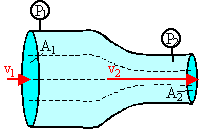Solution:

• Concepts:
Bernoulli's equation: P + ρgh + ½ρv2 = constant.
• Reasoning:
If we neglect friction, we have a conservative system.  Bernoulli's equation is derived assuming the mechanical energy of the system is conserved.  If a fluid or a gas, which is not being compressed, is flowing in a steady state, then the pressure depends on the speed of the fluid or the gas.  The faster the fluid is flowing, the lower is the pressure at the same height.
• Details of the calculation:
P1 + ρgh1 + ½ρv12 = P2 + ρgh2 + ½ρv22.
h is constant, so P1 + ½ρv12 = P2 + ½ρv22.
P1 - P2 = ½ρv22 - ½ρv12.
21 kPa = (350 kg/m3)(v22 - v12).
From the equation of continuity we have Area 1 * v1 = Area 2 * v2.
v1 = (A2/A1)v2.
Inserting this into the above equation we have(1 - (A2/A1)2)v22 = (21000/350)(m/s)2.
(A2/A1)2 = ¼2 = 1/16.  v22 = (21000/350)(16/15)(m/s)2 = 64 (m/s)2.
v2 = 8 m/s.
The fluid flow rate therefore is v2A2 = (8 m/s)π(0.01 m)2 = 0.0025 m3/s.

#### Problem:

Water circulates throughout a house in a hot-water heating system.  If the water is flowing at a speed of 0.2 m/s through a 5.0 cm-diameter pipe in the basement under a pressure of 3.0 atm, what will be the flow speed and pressure in a 1 cm-diameter pipe on the second floor 5.0 m above the basement?  Assume the pipes do not divide into branches. (1 atm = 101 kPa)

Solution:

• Concepts:
Bernoulli's equation: P + ρgh + ½ρv2 = constant
The equation of continuity: Area 1 * v1 = Area 2 * v2.
• Reasoning:
If we neglect friction, we have a conservative system.  Bernoulli's equation is derived assuming the mechanical energy of the system is conserved.  If a fluid or a gas, which is not being compressed, is flowing in a steady state, then the pressure depends on the speed of the fluid or the gas.  The faster the fluid is flowing, the lower is the pressure at the same height.
• Details of the calculation:
P1 + ρgh1 + ½ρv12 = P2 + ρgh2 + ½ρv22.
P2 = P1 + ρg(h1 - h2) + ½ρ(v12  - v22).
v2 = v1*25 = 5 m/s.
v12  - v22 = -24.96 (m/s)2.
P2 - P1  = -1000 kg/m3*9.8 m/s2*5 m - 500 kg/m3*24.96 (m/s)2  = -61480 Pa.
1 atmosphere = 101 kPa.
P2  = (3 - 0.61) atm = 2.39 atm = 241 kPa.